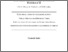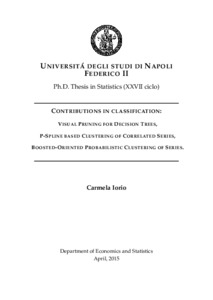Ricerca
Browse
Informazioni

Iorio, Carmela (2015) CONTRIBUTIONS IN CLASSIFICATION: VISUAL PRUNING FOR DECISION TREES, P-SPLINE BASED CLUSTERING OF CORRELATED SERIES, BOOSTED-ORIENTED PROBABILISTIC CLUSTERING OF SERIES. [Tesi di dottorato]Preview
Text
IorioCarmela_Thesis.pdf

[error in script] [error in script]
Item Type: Tesi di dottorato
Lingua: English
Title: CONTRIBUTIONS IN CLASSIFICATION: VISUAL PRUNING FOR DECISION TREES, P-SPLINE BASED CLUSTERING OF CORRELATED SERIES, BOOSTED-ORIENTED PROBABILISTIC CLUSTERING OF SERIES.
Creators:
CreatorsEmail
Iorio, Carmelacarmela.iorio@unina.it
Date: 30 March 2015
Number of Pages: 144
Institution: Università degli Studi di Napoli Federico II
Department: Scienze Economiche e Statistiche
Scuola di dottorato: Scienze economiche e statistiche
Dottorato: Statistica
Ciclo di dottorato: 27
Coordinatore del Corso di dottorato:
nomeemail
Lauro, Natale Carlonatale.lauro@unina.it
Tutor:
nomeemail
Siciliano, RobertaUNSPECIFIED
Aria, MassimoUNSPECIFIED
Date: 30 March 2015
Number of Pages: 144
Uncontrolled Keywords: Classification and Regression Trees, Cluster Analysis, P-Spline, Ensemble Methods.
Settori scientifico-disciplinari del MIUR: Area 13 - Scienze economiche e statistiche > SECS-S/01 - Statistica
Date Deposited: 13 Apr 2015 15:43
URI: http://www.fedoa.unina.it/id/eprint/10270
DOI: 10.6092/UNINA/FEDOA/10270## Abstract

This work consists of three papers written during my Ph.D. period. The thesis consists of five chapters. In chapter 2 the basic building blocks of our works are introduced. In particular we briefly recall the concepts of classification (supervised and unsupervised) and penalized spline. In chapter 3 we present a paper whose idea was presented at Cladag 2013 Symposium. Within the framework of recursive partitioning algorithms by tree-based methods, this paper provides a contribution on both the visual representation of the data partition in a geometrical space and the selection of the decision tree. In our visual approach the identification of both the best tree and of weakest links is immediately evaluable by the graphical analysis of the tree structure without considering the pruning sequence. The results in terms of error rate are really similar to the ones returned by the Classification And Regression Trees procedure, showing how this new way to select the best tree is a valid alternative to the well known cost-complexity pruning In chapter 4 we present a paper on parsimonious clustering of correlated series. Clustering of time series has become an important topic, motivated by the increased interest in these type of data. Most of the time, these procedures do not facilitate the removal of noise from data, have difficulties handling time series with unequal length and require a preprocessing step of the data considered, i.e. by modeling each series with an appropriate model for time series. In this work we propose a new clustering data (time) series way, which can be considered as belonging to both model-based and feature-based approach. Our method consists of since we model each series by penalized spline (P-spline) smoothers and performing clustering directly on spline coefficients. Using the P-spline smoothers the signal of series is separated from the noise, capturing the different shapes of series. The P-spline coefficients are close to the fitted curve and present the skeleton of the fit. Thus, summarizing each series by coefficients reduces the dimensionality of the problem, improving significantly computation time without reduction in performance of clustering procedure. To select the smoothing parameter we adopt a V-curve procedure. This criterion does not require the computation of the effective model dimension and it is insensitive to serial correlation in the noise around the trend. Using the P-spline smoothers, moments of the original data are conserved. This implies that mean and variance of the estimated series are equal to those of the raw series. This consideration allows to use a similar approach in dealing with series of different length. The performance is evaluated analyzing a simulated data set,also considering series with different length. An application of our proposal on financial time series is also performed. In Chapter 5 we present a paper that proposes a fuzzy clustering algorithm that is independent from the choice of the fuzzifier. It comes from two approaches, theoretically motivated for respectively unsupervised and supervised classification cases. The first is the Probabilistic Distance (PD) clustering procedure. The second is the well known Boosting philosophy. From the PD approach we took the idea of determining the probabilities of each series to any of the k clusters. As this probability is unequivocally related to the distance of each series from the cluster centers, there are no degrees of freedom in determine the membership matrix. From the Boosting approach we took the idea of weighting each series according some measure of badness of fit in order to define an unsupervised learning process based on a weighted re-sampling procedure. Our idea is to adapt the boosting philosophy to unsupervised learning problems, specially to non hierarchical cluster analysis. In such a case there not exists a target variable, but as the goal is to assign each instance (i.e. a series) of a data set to a cluster, we have a target instance. The representative instance of a given cluster (i.e. the center of a cluster) can be assumed as a target instance, a loss function to be minimized can be assumed as a synthetic index of the global performance, the probability of each series to belong to a given cluster can be assumed as the individual contribution of a given instance to the overall solution. In contrast to the boosting approach, the higher is the probability of a given series to be member of a given cluster, the higher is the weight of that instance in the re-sampling process. As a learner we use a P-spline smoother. To define the probabilities of each series to belong to a given cluster we use the PD clustering approach. This approach allows us to define a suitable loss function and, at the same time, to propose a fuzzy clustering procedure that does not depend on the definition of a fuzzifier parameter. The global performance of the proposed method is investigated by three experiments (one of them on simulated data and the remaining two on data sets known in literature) evaluated by using a fuzzy variant of the Rand Index. Chapter 6 concludes the thesis.